All submissions of the EM system will be redirected to Online Manuscript Submission System. Authors are requested to submit articles directly to Online Manuscript Submission System of respective journal.

# The Surprising Consequences of the ALF when Considered in the Context of Two Individually Moving Particles Coupled to Each Other

Ed Chen*, Tara Cronin

EM Sektet Hawaii, USA

*Corresponding Author:
Ed Chen
EM Sektet Hawaii, USA
E-mail: konarooster@gmail.com

Received: 05-Apr-2023, Manuscript No. JOMS-23-94341; Editor assigned: 07-Apr-2023, PreQC No. JOMS-23-94341 (PQ); Reviewed: 21-Apr-2023, QC No. JOMS-23-94341; Revised: 28-Apr-2023, Manuscript No. JOMS-23-94341 (R); Published: 05-May-2023, DOI: 10.4172/2321-6212.11.2.004.

Citation: Chen E, et al. The Surprising Consequences of the Abraham Lorentz Force (ALF) when Considered in the Context of Two Individually Moving Particles Coupled to Each Other. RRJ Mater Sci. 2023;11:004.

Copyright: © 2023 Chen E, et al. This is an open-access article distributed under the terms of the Creative Commons Attribution License, which permits unrestricted use, distribution, and reproduction in any medium, provided the original author and source are credited.

Visit for more related articles at Research & Reviews: Journal of Material Sciences

## Abstract

The Abraham Lorentz Force (ALF) is one of the basic forces discussed in electrodynamics and is the recoil force of a single charged particle emitting radiation under acceleration. The Abraham Lorentz force is a consequence of the conservation of momentum in a system of moving charged particles. This force has been relatively neglected in the literature, where most of the analysis has been limited to one particle systems. However, when the effects of the ALF are analyzed in the context of two particle interacting a surprising consequence arises: the Abraham Lorentz force, through induced magnetic emissions, will propagate through matter in a manner which is analogous to a compression wave that is transmitted from one recoiling particle triggering the adjacent particle through electromagnetic induction. This effect produces an attractive force between all interacting particles, and also predicts a sort of “supergravity” between matter and antimatter which causes them to attract each other at a greater rate than between matter and matter under certain conditions. This supergravity may be used to test the validity of the theory as applied to gravity

#### Keywords

Electrodynamics; Electromagnetic induction; Recoiling; Magnetic emissions; Electric field; Charged particle

#### Introduction

The Abraham Lorentz Force

The Abraham Lorentz force (ALF) is the recoil force of an accelerating charge in motion which acts in the direction opposite to the electromagnetic emission by the charged particle.

Traditionally it has been studied in the context of a dampening force that occurs when an electron interacts with its own electric field. Much of the literature discusses this force within the context of generalizing this force to quantum and relativistic contexts [1,2]. This force has also been discussed with respect to Unruh and Hawking radiation. All of these only examine the force in the context of one particle . This section will derive the equation for the ALF felt by each charged particle in a system of two individually moving but interacting charged particles.

AFL acts upon an electron when it emits electromagnetic radiation. It has been generalized into quantum mechanical terms by Dirac which eliminates certain anomalies such as runaway solutions and other problems where a charge will experience pre-acceleration before a force is applied. This also occurs in cases where the radius of the charge is less than 2/3 the radius of an electron. A relativistic quantum mechanical derivation has yet to be achieved . However, under certain reasonable assumptions about the electron, both pre-acceleration and run away solutions disappear . The net attractive effect of the ALF between two interacting charged particles is a basic exercise in physics which has been neglected to the detriment of modern physics.

#### Materials and Methods

In our model we will assume the electron is not a point charge, but has a radius. We will neither build a true one-dimensional classical model to avoid the problems of flux lines in one dimension which do not scale inversely with distance squared nor relativity models also fail for a 1 dimensional scheme. It is linear to simplify the model for the sake of ease of understanding .

When we examine the special case of two charged particles coupled together, with one active charge and one passive charge, we gain surprising insights into a consequence of this force that seems to mimic gravity. When we apply a spin to the particle at the origin, the spin will create a magnetic field that induces an electric field in the particle adjacent to it, which will induce a counter magnetic field. This counter magnetic field will oppose the initial magnetic field produce by the spin. At the moment the counter magnetic field is produced the coupled particles will jerk away from each other. At the moment of the jerk, the initial particle will emit energy in the direction opposite of the passive particle and recoil with an Abraham Lorentz force that is independent of the second particles (Figure 1).

Mathematically we can express this coupled recoil force. We begin with the Abraham-Lorentz radiative force equation: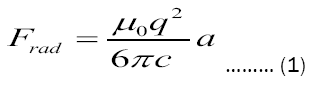At that moment, the Jerk felt by the origin particle can be described by the equation for magnetic force,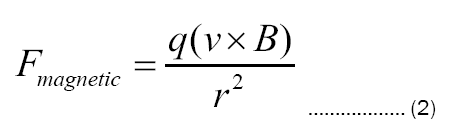Since the magnetic force will always be in the direction opposite of the passive particle, if we define the positive direction as the direction of the passive particle, then the magnetic force felt by the origin will always be negative, and away from the passive particle. The polarization of the charge will not matter with respect to the induced magnetic force. This is particularly interesting in explaining why matter and antimatter will feel gravity the same way if we neglect coulomb forces and in both cases will feel an attractive force from matter and antimatter (Figures 2 and 3).

Figure 2: shows the progression of the interaction of two charged particles, which were described by equations 1-8. We can see from this equation that only changes in the curl of the spin of the electron or changes in the magnetic field will result in a cancelation of the force. If we apply a large magnetic field in an attempt to cancel out the recoil force, we will only produce a larger recoil force since the Magnetic field will feed into B.

Figure 3: shows how a wave which originates with a single charged particle spinning would propagate through a row of charged particles causing each subsequent particle to recoil towards the origin.

We also know that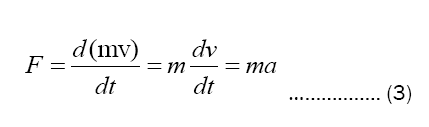To find the ALF felt by a single particle we need to find the acceleration, a, which is da/dt or the jerk of the active coil at the moment of analysis. Set Equation 2 equal to Equation 3 and we see that the acceleration of the system is Equation 2 divided by the mass. If we differentiate this equation, we get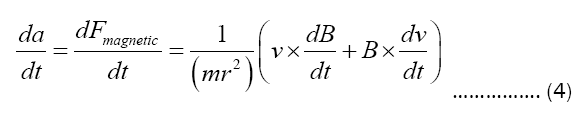Inserting Equation 4 into Equation 1 for da/dt gives us the equation for F-rad: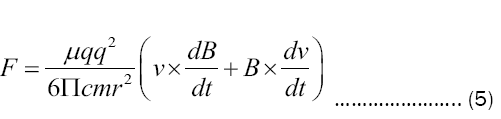We can also express equation 5 solely in terms of the magnetic field B.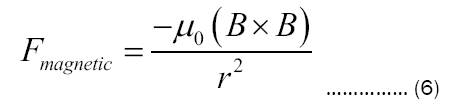Differentiating 6 with respect to time and dividing by mass gives us.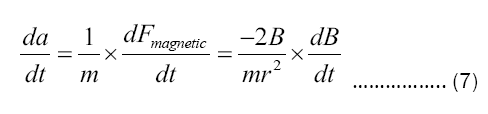If we take equation 4, which defines the acceleration of the particle, and set it equal to 0 so that the particle feels no force the equation becomes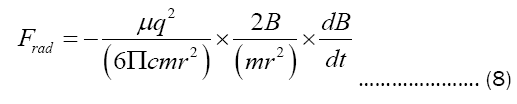#### Results and Discussion

Antimatter and matter

One place where the results of this derivation may be tested empirically is by testing the effect gravity has on antimatter and matter. Under general relativity, matter and antimatter are said to be equivalent and should respond identically to gravity [7,8]. Two types of experiments: antimatter free fall and antimatter beam charge to mass ratio have been used to detect the response of antimatter to gravity .

A particle bean experiment confirms the equivalence between matter and anti-matter to a less than 1% degree of uncertainty and confirms the Weak Equivalence Principle where the inertial and gravitational masses must be an identity. Further studies have shown the inertial mass to charge equivalence between matter and antimatter is greater than 1 part per billion . The scientific community is still awaiting the results of antimatter free fall as uncertainties continue to be ironed out .

When we add the coulomb emission and coulomb force into the model we find an interesting property where a charged particle pulse will create an additional attractive force when coupled with an oppositely charged particle though the magnetic spin will always induce attraction.

At the moment after the recoil jerk occurs due to the ALF acting on each of the particles, the charged particle will also emit an electric coulomb emission in the direction of the jerk, which will add or subtract to the initial jerk felt by the particles due to the Abraham Lorentz Force. The secondary jerks are induced both from coulomb forces from the first charged particle and due to the induced magnetic forces due to the induced spins. The energy emitted by the coulomb forces can be calculated with coulombs law [12-14].

During the recoil there will also be an additional Coulomb force that has to be factored in. Antimatter and matter will react differently due to the charge difference during the recoil phase where the particle feels the radiative force. If we assume anti-matter corresponds to a negatively charged particle while matter corresponds to a positively charged particle, we can see that mathematically, antimatter and matter will have an additional attractive force which predicts that “supergravity” will occur between matter and antimatter pairs under certain conditions. In this phase of the recoil, the antimatter and matter will follow the equation: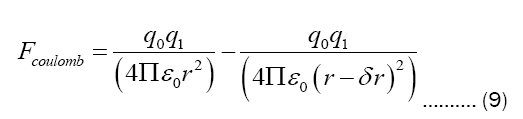In this case, dr will be the change in position felt by the particle due to the radiative force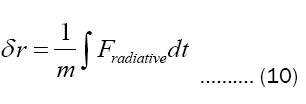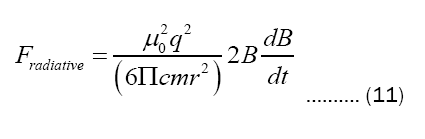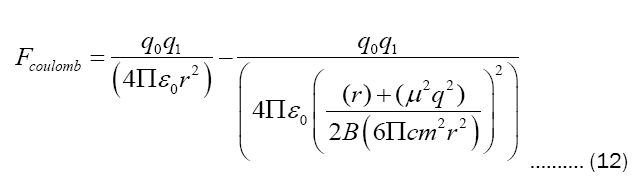When the particles are both matter pairs or anti-matter pairs (qo=q1), the coulomb force will always be positive in sign, signalling that the two particles will repel each other due to the coulomb force. On the other hand, when there is a matter and anti-matter particle in the system (q0=-q1) then the sign will also be negative, and the two particles will attract each other. There will actually be more attraction and thus more “gravity”. Due to the coulomb forces between oppositely charged particles during the recoil phase, two oppositely charged particles will feel an additional attractive force. We will observe a greater attraction between the matter and anti-matter. This is one aspect of the model which predicts behavior that we can verify by observation and which cannot be predicted by other models of gravity . Antimatter and matter should have the same charge to mass ratio as measured in a magnetic field. However, when antimatter freefall is measured in freefall, it should have a gravitational constant which is larger than matter.

However this effect only matters when r is on the same scale as the distance between the two charged particles. When r>> δr) the term vanishes. Furthermore, with respect to mass, we can also see that as the mass of the particle increases, r disappears.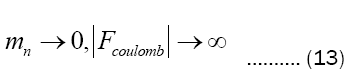Thus on an experimental level as the mass increases the coulomb term also disappears, which means that antimatter on large scales should display a very similar response to gravity as matter, although on a particle scale we should see deviations between matter and antimatter when the radius of separation is small and when the mass of the particles are very small. One prediction of this model is that gravity is produced at the subatomic level and therefore at the quantum level gravity will tend to disappear. This resolves the singularity issue in favor of quantum mechanics and disfavoring general relativity.

This model also elucidates the creation of electromagnetic compression waves which may be used to send communication signals through solid matter. Since all matter consists of agglomerations of charged particles we can easily see why all matter necessarily produces subatomic radiative force between any coupled particles. Gravity as described as a consequence of the radiative force would depend directly on the mass of the objects because mass is directly proportional to the number of charged particles in a system as protons and neutrons are both made from three spinning charged particles [16-18]. Gravity as a consequence of the radiative force would also depend inversely on distance between the objects because the compression wave is transmitted as a magnetic field inducing a current in an adjacent particle which then produces a subsequence magnetic field [19,20]. The field strength is inversely proportional. This also explains why all matter creates gravity and why anti matter still produces gravity despite its opposite spin.

#### Conclusion

The basic modeling of coupled Abraham Lorentz Force phenomenon will lead to a flowering of understanding in previously impenetrable fields. The theoretical foundations of electrodynamics will need to be further fleshed out for two body and multiple body consequences of the Abraham Lorentz Force. If confirmed, the recoil aspect of the force would generalizec to unify gravity as a consequence of electrodynamics. One aspect which may come into practical use will be Magnetic Compression Waves to transmit information. In theory, A signal can be embedded and transmitted through solid matter to receiver must like how a loud wave is transmitted and received electromagnetically. Using the generalized model for f-rad transmission for n-bodies through matter, we can see that electromagnetic compression waves can be used to carry signals through solid materials which transverse electromagnetic waves cannot penetrate. The use of the compression wave carriers will eliminate the need for satellite communications. These compression waves would essentially be gravity waves of different frequencies and amplitudes which are carried through matter by particles recoiling and readmitting a signal.

If the radiative force on a subatomic level seems to elucidate a connection between electromagnetism and gravity where gravity is the interaction of the radiative force between subatomic particles, thermodynamics may be the interaction between coupled electron shells at the molecular level. Thus as the energy of a system increases, coupled molecules will collide with increasing frequency due to increased recoiling between the molecules. When f-rad between the molecules is greater than the coulomb force of electrical repulsion a chemical reaction may occur. This also explains why some molecules such as chlorophyll are quantumly coupled to CO2. The frequencies of periodic recoils are arranged in some harmonic to each other so that the two molecules will always collapses towards each other. This insight may open the path for greater precision in computation chemistry as well as novel medical therapies.

While the recoil phenomenon between particles provides an interesting parallel to the force of gravity it does not fit cleanly with every aspect of the standard model of partially charged subatomic particles. If the magnetic compression waves are gravity waves, then neutrons and protons should have the same number of orbiting charges within them or the different charges within them would have to orbit as a faster velocity so equalize the mass of a proton and a neutron. Unfortunately, there is no explanation for why this would have to happen.

Furthermore, this model implies that particles have no rest mass, and that all mass occurs as a consequence of the Abraham Lorentz force on orbiting charges. This is another difficult assumption to swallow. Disregarding these uncomfortable implications, analyzing the consequences of the Abraham Lorentz force on coupled charged particles shows surprising consequences.